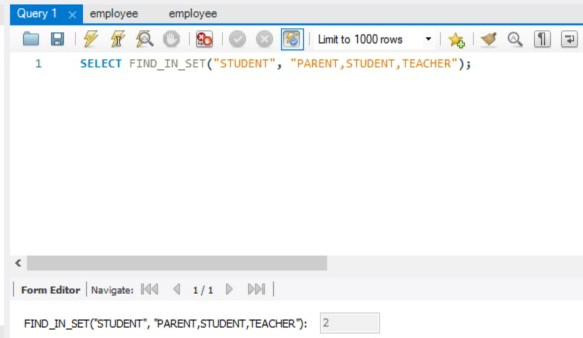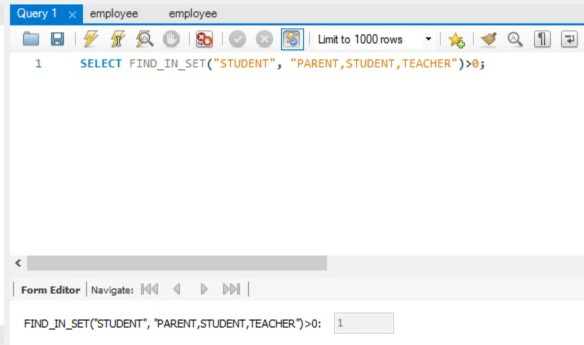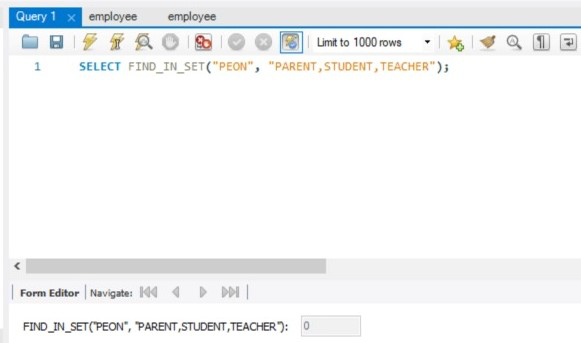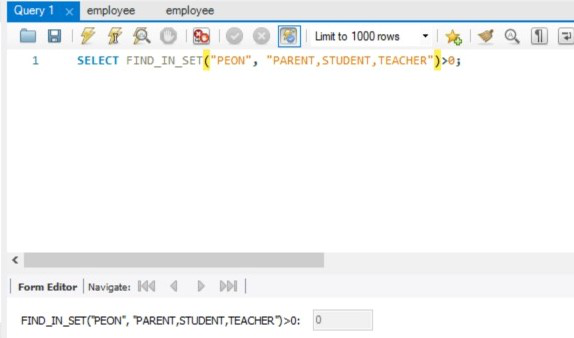Open in App
Not now

# Difference Between WHERE FIND_IN_SET(…)>0 and WHERE FIND_IN_SET(…) in SQL

• Last Updated : 30 Nov, 2021

FIND_IN_SET(…): This function returns the index(starting from 1) of the required string in a given list of strings if it is present in the list. If the required string is absent in the given list of strings, then 0 is returned.

Syntax:

```SELECT FIND_IN_SET(REQUIRED_STRING,
LIST_OF_STRINGS);```

FIND_IN_SET(…)>0: This function returns only 2 values i.e. either 1 or 0. 1 is returned if the required string is present in the given list of strings and 0 is returned if the required string is absent in the given list of strings.

Syntax:

```SELECT FIND_IN_SET(REQUIRED_STRING,
LIST_OF_STRINGS)>0;```

The following cases arise:

• When the REQUIRED_STRING is present in the given LIST_OF_STRINGS.

Query:

```SELECT FIND_IN_SET("STUDENT",
"PARENT,STUDENT,TEACHER");```

Output:Query:

```SELECT FIND_IN_SET("STUDENT",
"PARENT,STUDENT,TEACHER")>0;```

Output:• When the REQUIRED_STRING is absent in the given LIST_OF_STRINGS.

Query:

```SELECT FIND_IN_SET("PEON",
"PARENT,STUDENT,TEACHER");```

Output:Query:

```SELECT FIND_IN_SET("PEON",
"PARENT,STUDENT,TEACHER")>0;```

Output:When either the required string or the given list of strings is empty i.e. equal to “”, then 0 is returned by both the functions. Similarly, when either the required string or the given list of strings is NULL, then NULL is returned by both the functions

Thus, we can observe that the main difference between FIND_IN_SET(…) and FIND_IN_SET(…)>0 arises when the required string is present in the given list of strings. In this case, the FIND_IN_SET(…) function returns the index(starting from 1) of the required string whereas the FIND_IN_SET(…)>0 function returns 1 irrespective of the position of the required string.

My Personal Notes arrow_drop_up
Related Articles